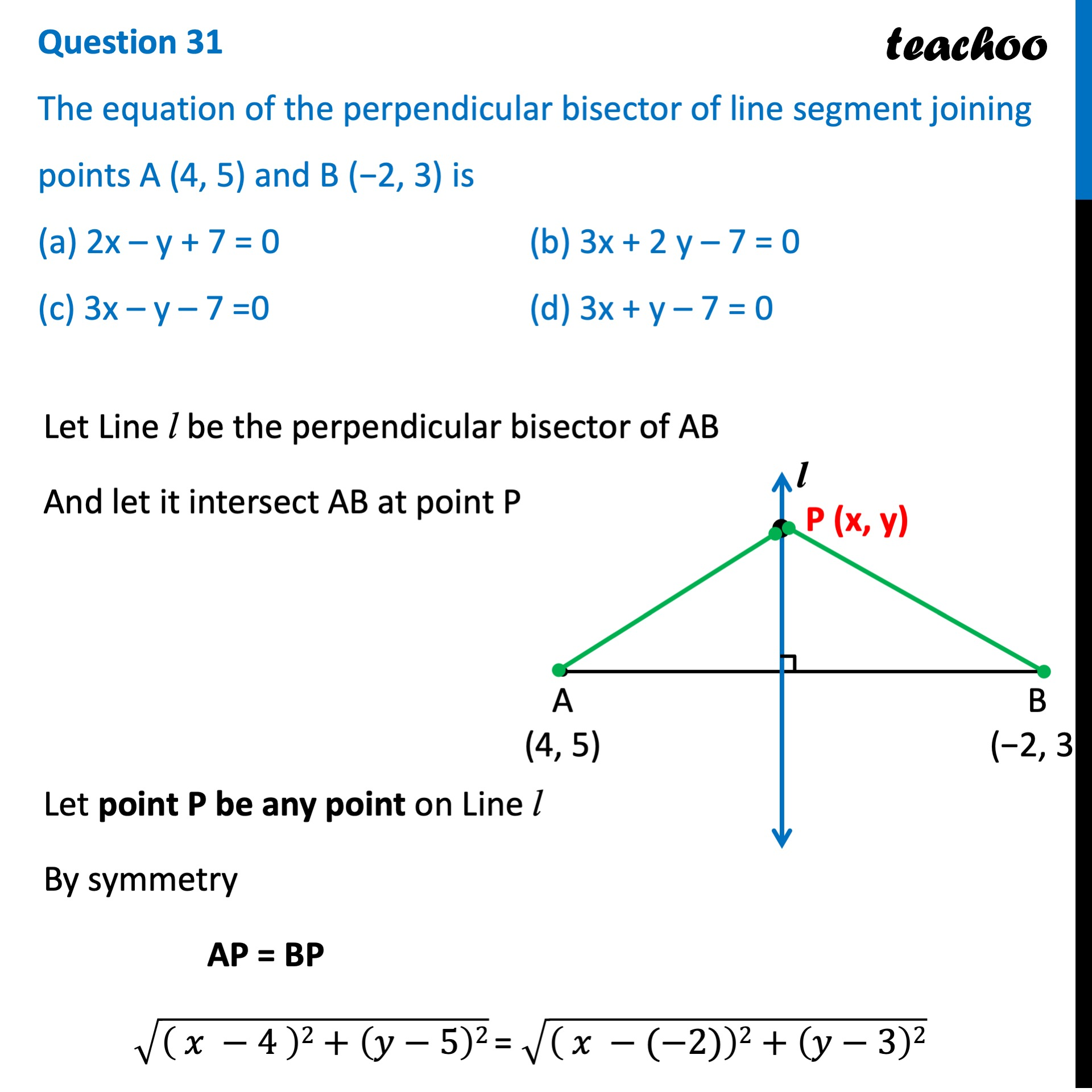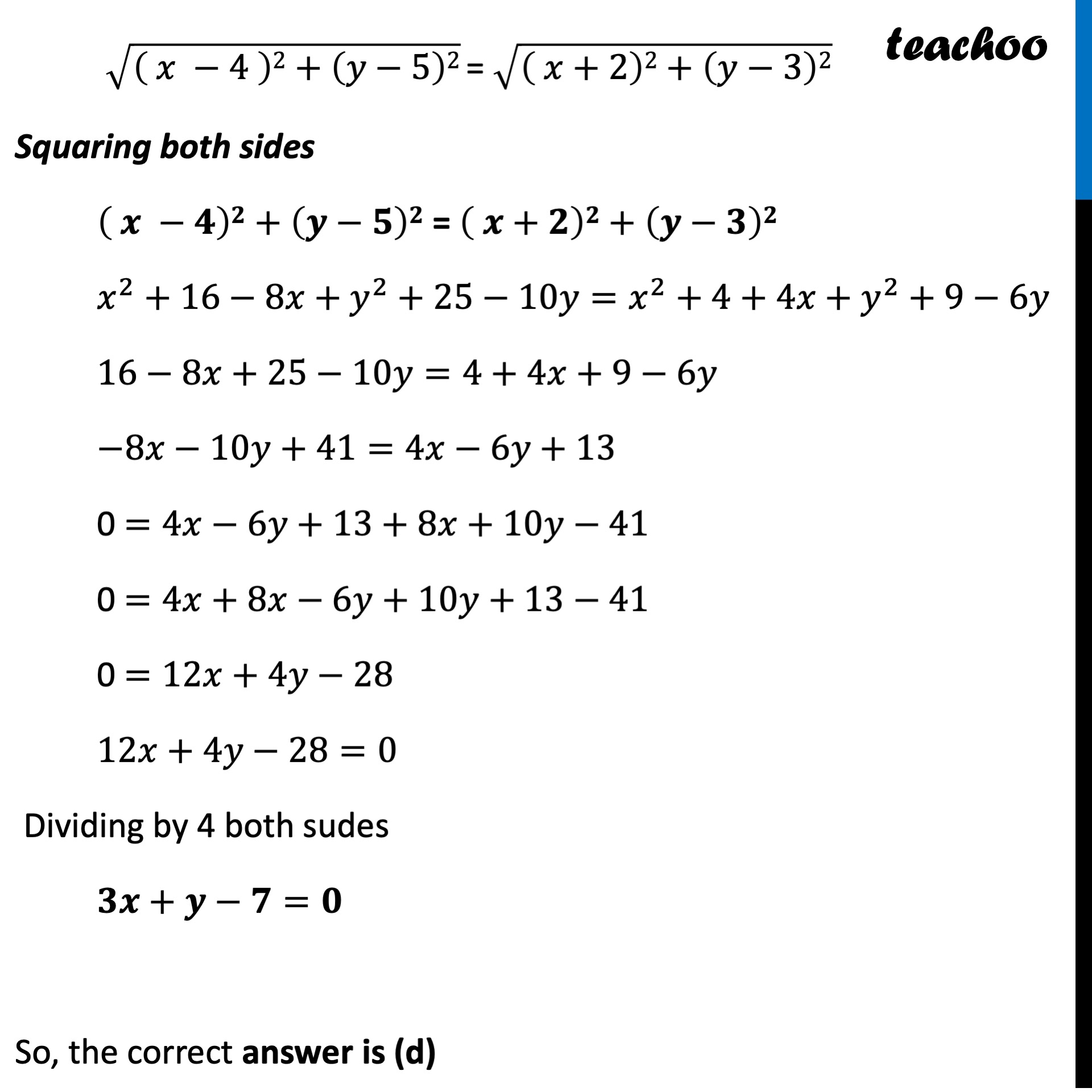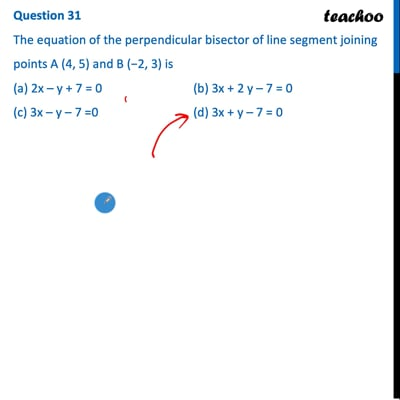CBSE Class 10 Sample Paper for 2022 Boards - Maths Standard [MCQ]

Class 10
Solutions of Sample Papers for Class 10 Boards

## (c) 3x – y – 7 =0       (d) 3x + y – 7 = 0This video is only available for Teachoo black users

Introducing your new favourite teacher - Teachoo Black, at only ₹83 per month

### Transcript

Question 31 The equation of the perpendicular bisector of line segment joining points A (4, 5) and B (−2, 3) is (a) 2x – y + 7 = 0 (b) 3x + 2 y – 7 = 0 (c) 3x – y – 7 =0 (d) 3x + y – 7 = 0 Let Line l be the perpendicular bisector of AB And let it intersect AB at point P Let point P be any point on Line l By symmetry AP = BP √(( 𝑥 −4 )2+(𝑦−5)2) = √(( 𝑥 −(−2))2+(𝑦−3)2) √(( 𝑥 −4 )2+(𝑦−5)2) = √(( 𝑥+2)2+(𝑦−3)2) Squaring both sides ( 𝒙 −𝟒)𝟐+(𝒚−𝟓)𝟐 = ( 𝒙+𝟐)𝟐+(𝒚−𝟑)𝟐 𝑥^2+16−8𝑥+𝑦^2+25−10𝑦=𝑥^2+4+4𝑥+𝑦^2+9−6𝑦 16−8𝑥+25−10𝑦=4+4𝑥+9−6𝑦 −8𝑥−10𝑦+41=4𝑥−6𝑦+13 0 =4𝑥−6𝑦+13+8𝑥+10𝑦−41 0 =4𝑥+8𝑥−6𝑦+10𝑦+13−41 0 =12𝑥+4𝑦−28 12𝑥+4𝑦−28=0 Dividing by 4 both sudes 𝟑𝒙+𝒚−𝟕=𝟎 So, the correct answer is (d)